## How to Calculate and Solve for Polar Moment of Inertia | Material Selection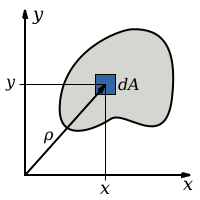The image above represents polar moment of inertia.

To compute for polar moment of inertia, one essential parameter is needed and this parameter is Radius (r).

The formula for calculating polar moment of inertia:

J = πr4/2

Where:

J = Polar Moment of Inertia

Let’s solve an example;
Find the polar moment of inertia when the radius is 22.

This implies that;

J = πr4/2
J = π(22)4/2
J = π(234256)/2
J = 735936.928/2
J = 367968.46

Therefore, the polar moment of inertia is 367968.46 m4.

## How to Calculate and Solve for Shear Stress | Material Selection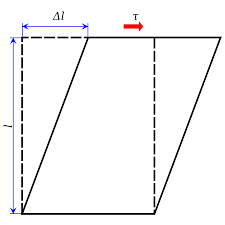The image above represents shear stress.

To compute for shear stress, three essential parameters are needed and these parameters are Twisting Moment (M), Radius (r) and Polar Moment of Inertia (J).

The formula for calculating shear stress:

τ = Mr/J

Where:

τ = Shear Stress
M = Twisting Moment
J = Polar Moment of Inertia

Let’s solve an example;
Find the shear stress when the twisting moment is 12, the radius is 8 and the polar moment of inertia is 14.

This implies that;

M = Twisting Moment = 12
J = Polar Moment of Inertia = 14

τ = Mr/J
τ = (12)(8)/14
τ = 96/14
τ = 6.85

Therefore, the shear stress is 6.85 Pa.

Calculating the Twisting Moment when the Shear Stress, the Radius and the Polar Moment of Inertia is Given.

M = τJ / r

Where;

M = Twisting Moment
τ = Shear Stress
J = Polar Moment of Inertia

Let’s solve an example;
Find the twisting moment when the shear stress is 10, the radius is 6 and the polar moment of inertia is 4.

This implies that;

τ = Shear Stress = 10
J = Polar Moment of Inertia = 4

M = τJ / r
M = 10 x 4 / 6
M = 40 / 6
M = 6.67

Therefore, the twisting moment is 6.67.

## How to Calculate and Solve for Acceleration | Motion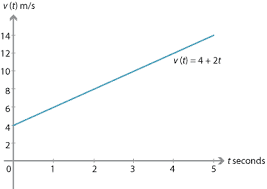The image above represents acceleration.

To compute for acceleration, two essential parameters are needed and these parameters are angular velocity (ω) and radius (r).

The formula for calculating acceleration:

a = ω²r

Where;

a = Acceleration
ω = Angular Velocity

Let’s solve an example;
Given that the angular velocity is 24 and the radius is 12. Find the acceleration?

This implies that;

ω = Angular Velocity = 24

a = ω²r
a = 24² x 12
a = 576 x 12
a = 6912

Therefore, the acceleration is 6912 m/s².

Calculating the Angular Velocity when the Acceleration and the Time is Given.

ω = √a / r

Where;

ω = Angular Velocity
a = Acceleration

Let’s solve an example;
Find the angular velocity when the acceleration is 50 with a radius of 2.

This implies that;

a = Acceleration = 50

ω = √a / r
ω = √50 / 2
ω = √25
ω = 5

Therefore, the angular velocity is 5.

## How to Calculate and Solve for Velocity | Motion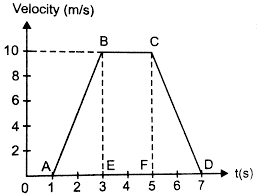The image above represents velocity.

To compute for velocity, two essential parameters are needed and these parameters are angular velocity (ω) and radius (r).

The formula for calculating velocity:

v = ωr

Where;

v = Velocity
ω = Angular Velocity

Let’s solve an example;
Find the velocity when the angular velocity is 17 with a radius of 6.

This implies that;

ω = Angular Velocity = 17

v = ωr
v = 17 x 6
v = 102

Therefore, the velocity is 102 m/s.

Calculating the Angular Velocity when the Velocity and the Radius is Given.

ω =v / r

Where;

ω = Angular Velocity
v = Velocity

Let’s solve an example;
Given that the velocity is 30 with a radius of 3. Find the angular velocity?

This implies that;

v = Velocity = 30

ω = v / r
ω = 30 / 3
ω = 10

Therefore, the angular velocity is 10.

## How to Calculate and Solve for Distance Covered | Motion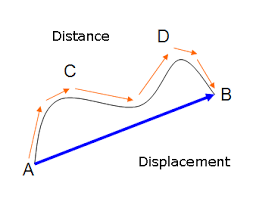The image above represents distance covered.

To compute for distance covered, two essential parameters are needed and these parameters are angular displacement (θ) and radius (r).

The formula for calculating distance covered:

S = rθ

Where;

S = Distance Covered
θ = Angular Displacement

Lets’s solve an example;
Find the distance covered when the angular displacement is 22 and the radius is 14.

This implies that;

θ = Angular Displacement = 22

S = rθ
S = 6 x 16
S = 96

Therefore, the distance covered is 96 m.

Calculating the Radius when the Distance Covered and the Angular Displacement is Given.

r = S / θ

Where;

S = Distance Covered
θ = Angular Displacement

Lets’s solve an example;
Find the radius when the distance covered is 70 and the angular displacement is 30.

This implies that;

S = Distance Covered = 70
θ = Angular Displacement = 30

r = S / θ
r = 70 / 30
r = 2.3

## How to Calculate and Solve for Road Bank Angle, Velocity and Radius of a Body in Motion of Circular Path | The Calculator Encyclopedia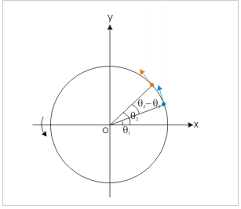The image represents road bank angle in circular motion.

To compute for the road bank angle, three essential parameters are needed and these parameters are velocity (v), acceleration due to gravity (g) and radius (r).

The formula for calculating the road bank angle;

θ = tan-1( / gr)

Where;
v = Velocity
g = Acceleration due to Gravity

Let’s solve an example;
Find the road bank angle where the acceleration due to gravity is 9.8, velocity is 35 and radius is 18.

This implies that;
v = Velocity = 35
g = Acceleration due to Gravity = 9.8

θ = tan-1( / gr)
θ = tan-1(35² / (9.8)(18))
θ = tan-1(1225 / 176.4)
θ = tan-1(6.94)
θ = 81.81°

Therefore, the road bank angle is 81.81°.

Calculating the Velocity when Road Bank Angle, Acceleration due to Gravity and Radius is Given.

v = √gr.tan θ

Where;
v = Velocity
g = Acceleration due to Gravity

Let’s solve an example;
Given that the road bank angle is 50, radius is 15 and acceleration due to gravity is 9.8. Find the velocity?

This implies that;
θ = Road Bank Angle = 50
g = Acceleration due to Gravity = 9.8

v = √gr.tan θ
v = √(9.8 x 15)(tan 50)
v = √(147)(1.1917)
v = √175.1799
v = 13.235

Therefore, the velocity is 13.235.

## How to Calculate and Solve for Centripetal Acceleration, Angular Velocity and Radius of Circular Path of a Body | The Calculator Encyclopedia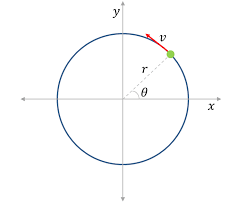The image above represents centripetal acceleration in a motion of circular path.

To compute for the centripetal acceleration, two essential parameters are needed and these parameters are angular velocity (ω) and radius of circular path (r).

The formula for the calculating centripetal acceleration:

a = ω²r

Where:
a = Centripetal Acceleration
ω = Angular Velocity
r = Radius of Circular Path

Let’s solve an example;
Find the centripetal acceleration with an angular velocity of 33 and a radius of 21.

This implies that;
ω = Angular Velocity = 33
r = Radius of Circular Path = 21

a = ω²r
a = 33² x 21
a = 1089 x 21
a = 22869

Therefore, the centripetal acceleration is 22869 m/s².

Calculating the Angular Velocity when Centripetal Acceleration and Radius of Circular path is Given.

ω = √a / r

Where:
ω = Angular Velocity
a = Centripetal Acceleration
r = Radius of Circular Path

Let’s solve an example;
Find the angular velocity with a centripetal acceleration of 320 and a radius of 8.

This implies that;
a = Centripetal Acceleration = 320
r = Radius of Circular Path = 8

ω = √a / r
ω = √320 / 8
ω = √40
ω = 6.32

Therefore, the angular velocity is 6.32.

## How to Calculate and Solve for Maximum Velocity, Coefficient of Friction and Radius of a Body in Motion of Circular Path | Nickzom Calculator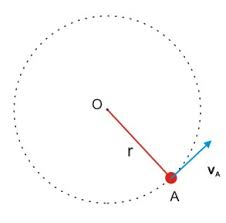The image above represents maximum velocity in circular motion.

To compute for the maximum velocity, three essential parameters are needed and these parameters are coefficient of friction (μ), radius (r) and acceleration due to gravity (g).

The formula for calculating maximum velocity:

Vmax = √(μgr)

Where;
Vmax = maximum velocity
μ = coefficient of friction
g = acceleration due to gravity

Let’s solve an example;
Find the maximum velocity when the coefficient of friction is 14 with a radius of 7 and acceleration due to gravity of 9.8.

This implies that;
μ = coefficient of friction = 14
g = acceleration due to gravity = 9.8

Vmax = √(μgr)
Vmax = √(14 x 7 x 9.8)
Vmax = √(960.40)
Vmax = 30.99

Therefore, the maximum velocity is 30.99 m/s.

Calculating the Coefficient of Friction when the Maximum Velocity, Radius and Acceleration due to Gravity is Given.

μ = Vmax2 / gr

Where;
μ = coefficient of friction
Vmax = maximum velocity
g = acceleration due to gravity

Let’s solve an example;
Find the coefficient of friction with a maximum velocity of 120, radius of 15 and acceleration due to gravity is 9.8?

This implies that;
Vmax = maximum velocity = 120
g = acceleration due to gravity = 9.8

μ = Vmax2 / gr
μ = 1202 / 15 x 9.8
μ = 14400 / 147
μ = 97.96

Therefore, the coefficient of friction is 97.96.

## How to Calculate and Solve for Superelevation, Guage of Track, Velocity and Radius of a Body in Circular Path Motion | The Calculator Encyclopedia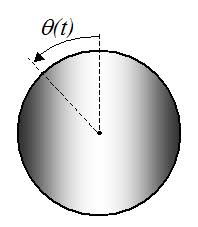The image above represents a body in superelevation.

To compute for the Superelevation, four essential parameters are needed and these parameters are Gauge of the track (G), velocity of the body (v), radius of the curve (r) and acceleration due to gravity (g).

The formula for calculating the circular path motion:

S = Gv² / gr

Where:
S = Superelevation
G = Gauge of the track
v = velocity of the body
r = radius of the curve
g = acceleration due to gravity

Let’s solve an example;
Find the circular path motion when Gauge of the track is 14, velocity of the track is 47, radius of the curve is 21 and acceleration due to gravity is 9.8.

This implies that;
G = Gauge of the track = 14
v = velocity of the body = 47
r = radius of the curve = 21
g = acceleration due to gravity = 9.8

S = Gv² / gr
S = 14 x 47² / 9.8 x 21
S = 14 x 2209 / 205.8
S = 30926 / 205.8
S = 150.27

Therefore, the superelevation is 150.27 m.

Calculating the Gauge of the track when Superelevation, Velocity of the body, Radius of the curve and Acceleration due to gravity.

G = Sgr / v2

Where;
G = Gauge of the track
S = Superelevation
v = velocity of the body
r = radius of the curve
g = acceleration due to gravity

Let’s solve an example;
With a superelevation of 180, velocity of the body is 32, radius of the curve is 12 and acceleration due to gravity as 9.8. Find the gauge of the track?

This implies that;
S = Superelevation = 180
v = velocity of the body = 32
r = radius of the curve = 12
g = acceleration due to gravity = 9.8

G = Sgr / v2
G = 180 x 12 x 9.8 / 322
G = 21168 / 1024
G = 20.67

Therefore, the gauge of the track is 20.67.

Calculating the Velocity of the body when Superelevation, Gauge of the track, Radius of the curve and Acceleration due to gravity.

v = √Sgr / G

Where;
v = velocity of the body
S = Superelevation
G = Gauge of the track
r = radius of the curve
g = acceleration due to gravity

Let’s solve an example;
With a superelevation of 120, gauge of the track is 28, radius of the curve is 7 and acceleration due to gravity as 9.8. Find the velocity of the body?

This implies that;
S = Superelevation = 120
G = gauge of the track = 28
r = radius of the curve = 7
g = acceleration due to gravity = 9.8

v = √Sgr / G
v = √120 x 9.8 x 7 / 28
v = √8232 / 28
v = √294
v = 17.146

Therefore, the velocity of the body is 17.146.

## How to Calculate and Solve for Mass, Angular Velocity, Radius and Centrifugal Force of a Body | The Calculator Encyclopedia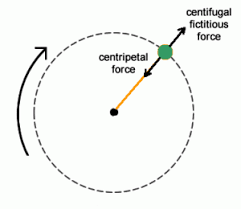The image above represents the centrifugal force.

To compute for the centrifugal force, three essential parameters are needed and these parameters are Mass of the body (m), Angular Velocity of the body (w) and Radius (r).

The formula for calculating the centrifugal force:

F = mω²r

Where:
F = Centrifugal Force
m = mass of the body
ω = angular velocity

Let’s solve an example;
Find the centrifugal force with mass of the body as 12, angular velocity as 32 and a radius of 8.

This implies that;
m = mass of the body = 12
ω = angular velocity = 32

F = mω²r
F = 12 x 32² x 8
F = 12 x 1024 x 8
F = 98304

Therefore, the centrifugal force is 98304 N.

Calculating the Mass of the body (m) when the Centrifugal Force, Angular Velocity and Radius is Given.

m = F / w2r

Where;
m = mass of the body
F = Centrifugal Force
ω = angular velocity

Let’s solve an example;
Find the mass of a body when centrifugal force is 140 with an angular velocity of 24 and a radius of 10.

This implies that;
F = Centrifugal Force = 140
ω = angular velocity = 24